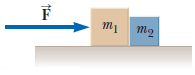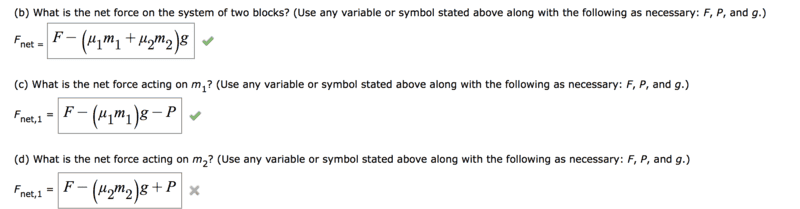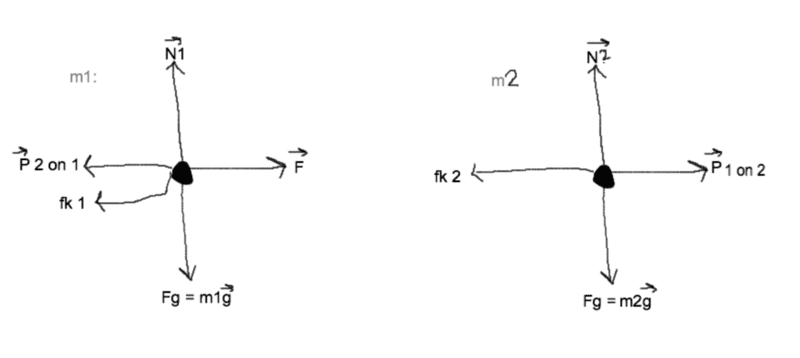# A question about contact forces and friction

## Homework Statement

Two blocks of masses m1 and m2 are placed on a table in contact with each other as shown in the figure below. The coefficient of kinetic friction between the block of mass m1 and the table is μ1, and that between the block of mass m2 and the table is μ2. A horizontal force of magnitude F is applied to the block of mass m1. We wish to find P, the magnitude of the contact force between the blocks.## Homework Equations

F = ma
Kinetic force = μk x FN

## The Attempt at a Solution

I was pretty sure my answer for (d) was right. I'm not sure what I did wrong[/B]#### Attachments

gneill
Mentor
Draw a free-body diagram for ##m_2##. What forces are directly acting on ##m_2##?

Mister T
Gold Member
I was pretty sure my answer for (d) was right. I'm not sure what I did wrong
You would have to tell us what you did before we can tell you what you did wrong. Can you tell us what you were thinking when you were "pretty sure" you'd done it correctly?

Note, by the way, that ##P## is an internal force.

jbriggs444
Homework Helper
Note, by the way, that ##P## is an internal force.
Whether a force is "internal", "external", or irrelevant depends on where you have drawn the boundaries around the system of interest. For question d, I would draw the boundaries such that P is an external force.

You would have to tell us what you did before we can tell you what you did wrong. Can you tell us what you were thinking when you were "pretty sure" you'd done it correctly?

Note, by the way, that ##P## is an internal force.That's my free body diagram

For m1:

Fnet = F - P - μ1(N) ---> F - (μ1m1)g - P

For m2: (I'm not sure if F is still included in this equation or not?)

Fnet = F + P - μ2(N) ---> F - (μ2m2)g + P (this answer was wrong)

#### Attachments

Whether a force is "internal", "external", or irrelevant depends on where you have drawn the boundaries around the system of interest. For question d, I would draw the boundaries such that P is an external force.

Is my free body diagram correct?jbriggs444